#### Chapter 17 Construction R.D. Sharma Solutions for Class 9th Exercise 17.1

Exercise 17.1

1Draw a line segment of length 8.6 cm. Bisect it and measure the length of each part.

Solution

We are given a line segment of length 8.6 cm
We have to bisect it and measure each part.We follow the following steps of construction

STEP 1: Draw a line segment AB = 8.6 cm by using a graduated ruler.

STEP 2: With A as centre and radius more than half of AB, draw arcs, one on each side of AB.

STEP 3: With B as centre and the same radius as in step II, draw arcs cutting the arcs drawn in step II at E and F respectively.

STEP 4: Draw the line segment with E and F as end-points. Suppose it meets AB at M. Then M bisects the line segment AB.
By measuring AM and MB, we find that
AM = MB = 4.3cm

2. Draw a line segment AB of length 5.8 cm. Draw the perpendicular bisector of this line segment.

Solution

We are given the line segment of length 5.8 cm
We are asked to draw the perpendicular bisector of the line segment
We will follow the following algorithm for the construction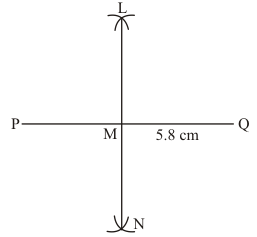We follow the following steps for constructing the perpendicular bisector of PQ.

STEP 1: Draw a line segment PQ=5.8 cm by using a graduated ruler.

STEP 2: With P as centre and radius more than half of PQ, draw two arcs, one on each side of PQ.

STEP 3: With Q as centre and the same radius as in step II, draw arcs cutting the arcs drawn in the previous step at Land N respectively.

STEP 4: Draw the line segment with L and N end-points.
The line segment LN is the required perpendicular bisector of Q.

3. Draw a circle with centre at point O and radius 5 cm. Draw its chord AB, draw the perpendicular bisector of line segment AB. Does it pass through the centre of the circle?

Solution

We are asked to draw the circle centered at O of radius 5 cm with its chord AB
We will follow the following algorithm for the construction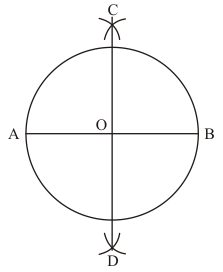STEP 1: Draw a circle with centre at point O and radius 5 cm.

STEP 2: Draw its cord AB.

STEP 3: With A as centre and radius more than half of AB, draw two arcs, one on each side of AB.

STEP 4: With B as centre and the same radius as in step3, draw arcs cutting the arcs drawn in the previous step at C and D respectively.

STEP 5: Draw the line segment with C and D as end-points. The line segment CD is the required perpendicular bisector of AB. Since CD is perpendicular bisector of AB which is chord of circle, hence it passes through the centre of the circle.

4. Draw a circle with centre at point O. Draw its two chords AB and CD such that AB is not parallel to CD. Draw the perpendicular bisectors of AB and CD. At what point do they intersect ?

Solution

We are asked to draw a circle centered at O and two chords AB and CD
We will follow the following algorithm for the constructionSteps of construction

STEP 1: With centre O, draw a circle of any radius.

STEP 2: Draw any two chords AB and CD, such that the two chords are not parallel.

STEP 3: With centre B and taking any radius (more than half of AB), draw two arcs, one on each side of the chord AB.

STEP 4: With centre A, and taking the same radius, draw two arcs, one on each side of the chord AB, cutting the previous arcs in E and F respectively.

STEP 5: Draw a line segment with E and F as end-points. It passes through centre O.

STEP 6: With centre C and taking any radius (more than half of CD), draw two arcs, one on each side of the chord CD.

STEP 7: With centre D, and taking the same radius as in STEP 6, draw two arcs, one on each side of the chord CD, cutting the previous arcs in G and H respectively.

STEP 8: Draw a line segment with G and H as end-points. This also passes through centre O. It is clear that perpendicular bisectors EF and GH intersect at point O, which is the centre of the circle.

5. Draw a line segment of length 10 cm and bisect it. Further bisect one of the equal parts and measure its length.

Solution

We are asked to bisect the line segment of length 10 cm and again bisect the half of its original line segment .
We follow the following steps for the construction .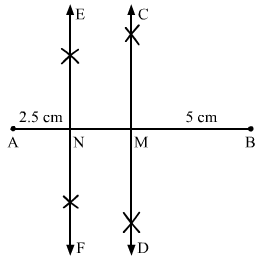Steps of construction

STEP 1: Draw line segment AB having length 10 cm.

STEP 2: With centre A and radius greater than half of AB, draw two arcs one on each side of AB.

STEP 3: With centre B and taking same radius, draw two arcs one on each side of AB, intersecting the previous two arcs at C and D respectively.

STEP 4: Draw a line segment having end-points C and D. Segment CD is the perpendicular bisector of AB. Let CD intersects AB at M.

STEP 5: with centre A and radius greater than half of AM, draw two arcs one on each side of AM.

STEP 6: With centre M and taking same radius, draw two arcs one on each side of AM intersecting the previous two arcs at E and F respectively.

STEP 7: Draw a line segment having end-points E and F. Segment EF is the perpendicular bisector of AM. Let EF intersects AM at N.

STEP 8: Measure the length of AN.
Length of line segment AN must be 2.5 cm

6. Draw a line segment AB and bisect it. Bisect one of the equal parts to obtain a line segment of length 1/2 (AB).

Solution

We are asked to draw a line segment of any length, bisect it and again bisect it such that it is equals to 1/4 (AB)
We will follow certain algorithm to construct the figure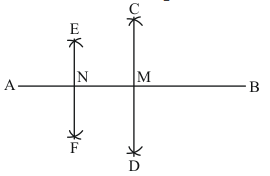Steps of construction

STEP 1: Draw line segment AB of any length.

STEP 2: With centre A and radius greater than half of AB, draw two arcs one on each side of AB.

STEP 3: With centre B and taking same radius, draw two arcs one on each side of AB, intersecting the previous two arcs at C and D respectively.

STEP 4: Draw a line segment having end-points C and D. Segment CD is the perpendicular bisector of AB. Let CD intersects AB at M.

STEP 5: With centre A and radius greater than half of AM, draw two arcs one on each side of AM.

STEP 6: With centre M and taking same radius, draw two arcs one on each side of AM, intersecting the previous two arcs at E and F respectively.

STEP 7: Draw a line segment having end-points E and F. Segment EF is the perpendicular bisector of AM. Let EF intersects AM at N.
AN = 1/4(AB).

Disclaimer : In the question, instead of 1/2 (AB), there should be 1/4 (AB).

7. Draw a line segment AB and by ruler and compasses, obtain a line segment of length 3/4 (AB).

Solution

We are asked to draw a line segment AB of any length and get a line segment of
Length  3/4(AB)
We will follow the following algorithm for the construction .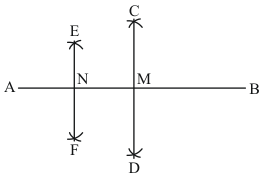Steps of construction

STEP 1: Draw line segment AB of any length.

STEP 2: With centre A and radius greater than half of AB, draw two arcs one on each side of AB.

STEP 3: With centre B and taking same radius, draw two arcs one on each side of AB, intersecting the previous two arcs at C and D respectively.

STEP 4: Draw a line segment having end-points C and D. Segment CD is the perpendicular bisector of AB. Let CD intersects AB at M.

STEP 5: With centre A and radius greater than half of AM, draw two arcs one on each side of AM.

STEP 6: With centre M and taking same radius, draw two arcs one on each side of AM, intersecting the previous two arcs at E and F respectively.

STEP 7: Draw a line segment having end-points E and F. Segment EF is the perpendicular bisector of AM. Let EF intersects AM at N
Here, NB = 3/4(AB) .